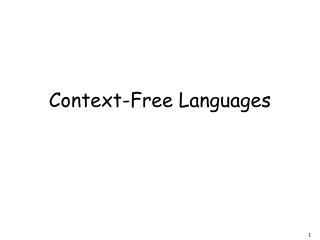DownloadDownload PresentationContext-Free Languages

# Context-Free Languages

Download Presentation## Context-Free Languages

- - - - - - - - - - - - - - - - - - - - - - - - - - - E N D - - - - - - - - - - - - - - - - - - - - - - - - - - -
##### Presentation Transcript

1. Context-Free Languages

2. Regular Languages

3. Context-Free Languages Regular Languages

4. stack automaton Context-Free Languages Context-Free Grammars Pushdown Automata

5. Context-Free Grammars

6. Grammars • Grammars express languages • Example: the English language

7. A derivation of “the dog walks”:

8. A derivation of “a cat runs”:

9. Language of the grammar: L = { “a cat runs”, “a cat walks”, “the cat runs”, “the cat walks”, “a dog runs”, “a dog walks”, “the dog runs”, “the dog walks” }

10. Notation Production Rules Variable Terminal

11. Another Example • Grammar: • Derivation of sentence :

12. Grammar: • Derivation of sentence :

13. Other derivations:

14. Language of the grammar

15. More Notation • Grammar Set of variables Set of terminal symbols Start variable Set of Production rules

16. Example • Grammar :

17. More Notation • Sentential Form: • A sentence that contains • variables and terminals • Example: Sentential Forms sentence

18. We write: • Instead of:

19. In general we write: • If:

20. By default:

21. Example Grammar Derivations

22. Example Grammar Derivations

23. Another Grammar Example • Grammar : Derivations:

24. More Derivations

25. Language of a Grammar • For a grammar • with start variable : String of terminals

26. Example • For grammar : Since:

27. A Convenient Notation

28. Example A context-free grammar : A derivation:

29. A context-free grammar : Another derivation:

30. (((( )))) Describes parentheses:

31. Example A context-free grammar : A derivation:

32. A context-free grammar : Another derivation:

33. Example A context-free grammar : A derivation:

34. A context-free grammar : A derivation:

35. Describes matched parentheses: () ((( ))) (( ))

36. Definition: Context-Free Grammars Grammar Variables Terminal symbols Start variable Productions of the form: Variable String of variables and terminals

37. Definition: Context-Free Languages • A language is context-free • if and only if • there is a context-freegrammar • with

38. Leftmost derivation: Rightmost derivation: Derivation Order

39. Leftmost derivation: Rightmost derivation:

40. Derivation Trees

41. Derivation Tree

42. Derivation Tree yield

43. Partial Derivation Trees Partial derivation tree